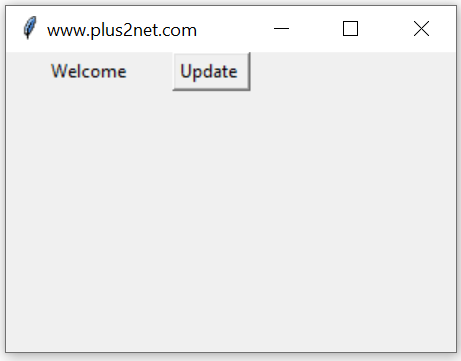# Python tkinter StringVar() trace method

``str1=tk.StringVar(master,value,name)``
`master`: (Optional)The variable is associated with, default value is parent window.
`value`:(Optional) We can set the initial value for the variable.
`name` : (Optional) Name given default is PY_VAR1

Tkitner StringVar() get(), set(), trace() methods to manage data and trigger call back functions

For an StringVar() so we can check the different modes of this variable and trigger call back functions. This is the main advantage of using such variables.

w :Write- the variable is written ( updated ) by someone ( frequently used).
u :undefined – The variable is deleted

## Example of uses of StringVarWe used one Button and on Click event of this we change the value of the String variable str1 from Hello to Welcome.
``b1 = tk.Button(my_w,text='Update',command=lambda:str1.set('Welcome') )``
This triggers the trace() which used the callback function my_r() to print the value of the variable ( Welcome ) to our console.
``````import tkinter as tk
from tkinter import *
my_w = tk.Tk()
my_w.geometry("300x200")  # Size of the window

def my_r(*args):
print(str1.get()) #  Print when variable changes.

str1 = tk.StringVar(my_w) # declare StringVar()
str1.set('Hello')

l3 = tk.Label(my_w,  textvariable=str1, width=15 )
l3.grid(row=2,column=1)

b1 = tk.Button(my_w,text='Update',command=lambda:str1.set('Welcome'))
b1.grid(row=2,column=3)

str1.trace('w',my_r)

my_w.mainloop() ``````
Output
``Welcome``

## set() and get() methods of StringVar

In above examples we used set() method to assign data to the string variable and to read the data stored we used get() method. These two methods are frequently used in our scripts.
``````str1.set('Hello') # assign value to str1
print(str1.get()) # display the value assigned to str1``````

## Initializing StringVar

We can using set() method to assign data to StringVar after declaring or we can assign value while declaring the string variable.
``str1=tk.StringVar(value='Option 1') # Assign value to str1``

## Length of StringVar

We can use len
``len(str1.get())``

## Normal Variable and StringVar

StringVar() is a class in Tkinter. In a GUI application we require various events to be captured to trigger different functions (requirements). If we use normal Python variable with widgets then monitoring the changes is difficult. However by using a StringVar we can monitor the changes and trigger functions based on the requirements.

Example : Declare the password is strong or weak based on the number of chars entered by user. Here we can use StringVar and each time the variable changes its stored data, we can trigger the function checking the length of the input and show the message.

## More Examples on StringVar()

The most frequently used method is trace(), by using this we can track the changes and trigger function. Here are some scripts using trace() method of StringVar().

## Counting the number of charsAs we enter data inside the entry box, the total number of chars entered will be displayed by the side ( Label l1 ) . Here StringVar() e1_str is connected to Entry widget e1 using textvariable option.

The trace() method of e1_str is used to trigger the function my_upd() whenever the e1_str data changes.

Inside the function my_upd() we read the user input by using e1.get() , then found out the number of chars by using len() and the converted the integer to string by using str() function.
``````import tkinter as tk
my_w = tk.Tk()
from tkinter import *
my_w.geometry("400x150")  # Size of the window width x height

e1_str=tk.StringVar() # declaring a StringVar()
e1 = tk.Entry(my_w,textvariable=e1_str,bg='yellow',font=28) # Entry box

l1 = tk.Label(my_w,  text='No of Chars here' ,font=28)  # added one Label
l1.grid(row=0,column=1)

def my_upd(*args):
l1.config(text=str(len(e1_str.get()))) # read & assign text to StringVar()

e1_str.trace('w',my_upd) # triggers on change of StringVar
my_w.mainloop()``````

Subscribe to our YouTube Channel here

## Subscribe

* indicates required
Subscribe to plus2netplus2net.com

 22-03-2021 Very bad to call a variable in Python "str" str = tk.StringVar(my_w) # declare StringVar() because str is build-in method to stringify data! class str(object='') --> built-in function in Python
 23-03-2021 Thanks, Let us use str1